# 从锦囊妙计想到的22–逐步细化的办法解决复杂问题

categories:儿童计算机  author:

19中， 介绍了一下计算机的输入和输出系统， 既然说到了输出必不可少的 需要输入来对应， 那么有几种输入方法， 有哪些设备是输入设备， 哪些是输出设备。 在输入是为什么要回显！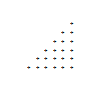# 一。 分析输出的内容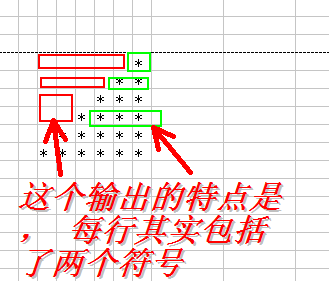# 二。 编写程序实现说明

f1空白（）输出f1行的空白部分， f1星（）输出*的部分，类似

f1空白，f2星（），。。。。。。等

# 三。采用java实现上述的程序```package cn.iigrowing.java.study;
public class TuXingShuChu {
public static void main(String[] args) {
f1();
f2();
f3();
f4();
f5();
f6();
}
// 输出第一行
public static void f1(){
f1kongBai();  // 输出空白
f1Xing();     // 输出*
System.out.println();  // 输出换行
}
// 输出第一行的 空白
public static void f1kongBai()
{
}
// 输出第一行的 *
public static void f1Xing()
{
}
}
``````package cn.iigrowing.java.study;
public class TuXingShuChu2 {
public static void main(String[] args) {
f1(); // 输出第一行
f2(); // 输出第二行
f3(); // 输出第三行
f4(); // 输出第四行
f5(); // 输出第五行
f6(); // 输出第六行
}
// 输出第一行
public static void f1(){
kongbai(5);  // 输出空白
xing(1);     // 输出*
System.out.println();  // 输出换行
}
public static void f2(){
kongbai(4);  // 输出空白
xing(2);     // 输出*
System.out.println();  // 输出换行
}
public static void f3(){
kongbai(3);  // 输出空白
xing(3);     // 输出*
System.out.println();  // 输出换行
}

public static void f4(){
kongbai(2);  // 输出空白
xing(4);     // 输出*
System.out.println();  // 输出换行
}

public static void f5(){
kongbai(1);  // 输出空白
xing(5);     // 输出*
System.out.println();  // 输出换行
}

public static void f6(){
kongbai(0);  // 输出空白
xing(6);     // 输出*
System.out.println();  // 输出换行
}
// 输出一行 空白
public static void kongbai(int count)
{
for(int i = 0; i < count; i++){
System.out.print(" ");
}
}
// 输出一行*
public static void xing(int count)
{
for(int i = 0; i < count; i++){
System.out.print("*");
}
}
}
```

# 四。 优化调整代码逻辑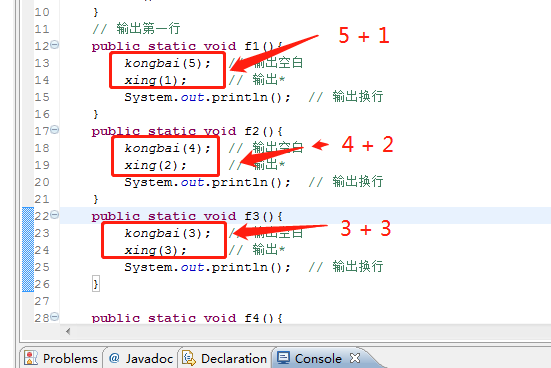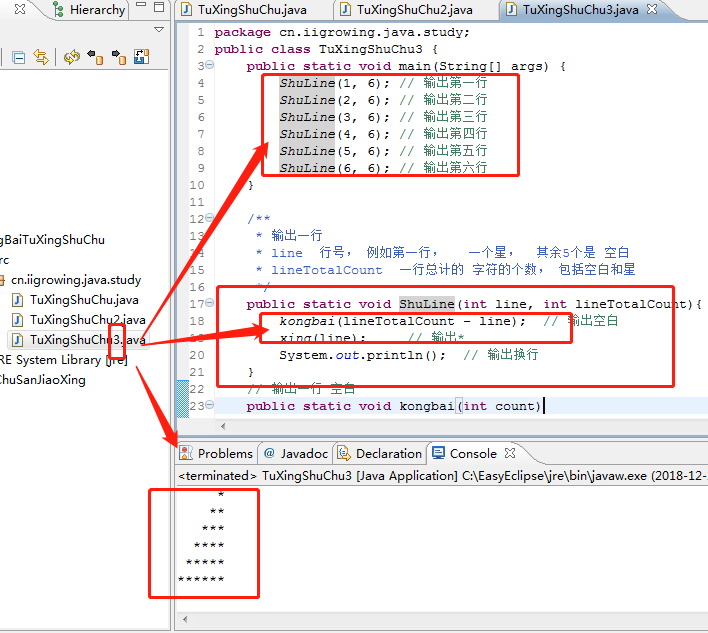```package cn.iigrowing.java.study;
public class TuXingShuChu3 {
public static void main(String[] args) {
ShuLine(1, 6); // 输出第一行
ShuLine(2, 6); // 输出第二行
ShuLine(3, 6); // 输出第三行
ShuLine(4, 6); // 输出第四行
ShuLine(5, 6); // 输出第五行
ShuLine(6, 6); // 输出第六行
}

/**
* 输出一行
* line  行号， 例如第一行，   一个星，  其余5个是 空白
* lineTotalCount  一行总计的 字符的个数， 包括空白和星
*/
public static void ShuLine(int line, int lineTotalCount){
kongbai(lineTotalCount - line);  // 输出空白
xing(line);     // 输出*
System.out.println();  // 输出换行
}
// 输出一行 空白
public static void kongbai(int count)
{
for(int i = 0; i < count; i++){
System.out.print(" ");
}
}
// 输出一行*
public static void xing(int count)
{
for(int i = 0; i < count; i++){
System.out.print("*");
}
}
}
```

# 五。再次优化为实用版本```package cn.iigrowing.java.study;
public class TuXingShuChu6 {
public static void main(String[] args) {
final int lineTotalCount = 6;   // 定义一个  每行的最多字符数， 同时也是 行数，
for(int i = 1; i <= lineTotalCount; i++)
{
ShuLine(i, lineTotalCount); // 输出第 i 行
}
}
/**
* 输出一行
* line  行号， 例如第一行，   一个星，  其余5个是 空白
* lineTotalCount  一行总计的 字符的个数， 包括空白和星
*/
public static void ShuLine(int line, int lineTotalCount){
kongbai(lineTotalCount - line);  // 输出空白
xing(line);     // 输出*
System.out.println();  // 输出换行
}
// 输出一行 空白
public static void kongbai(int count)
{
for(int i = 0; i < count; i++){
System.out.print(" ");
}
}
// 输出一行*
public static void xing(int count)
{
for(int i = 0; i < count; i++){
System.out.print("*");
}
}
}
```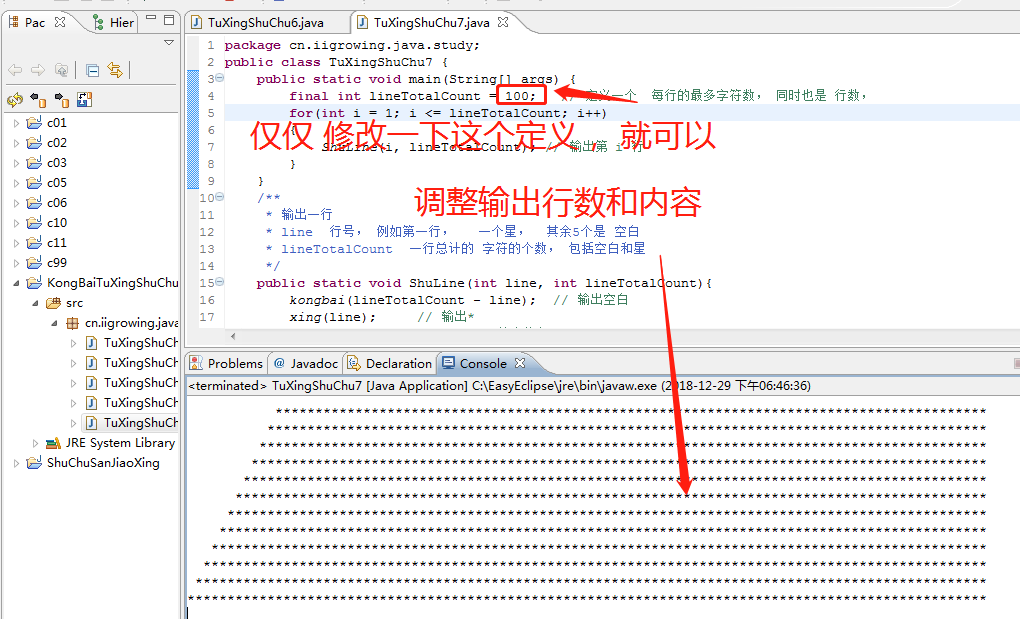# 六。 程序的流程图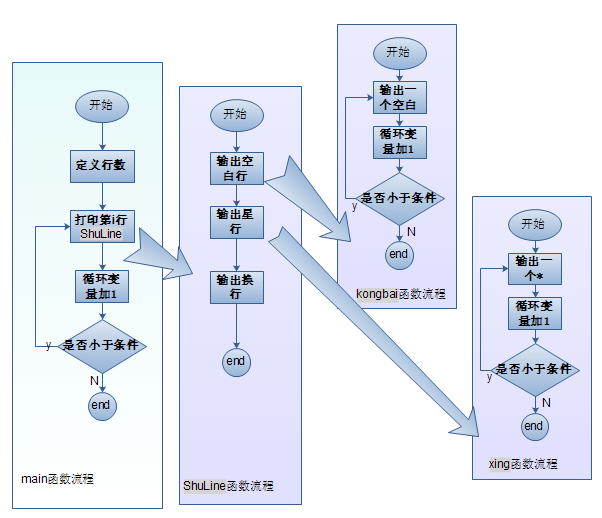cpu和线程定义、开始               从锦囊妙计想到的07

java函数控制输出                     从锦囊妙计想到的21

java入门                                    从锦囊妙计想到的23

java复杂过程分析                   从锦囊妙计想到的25